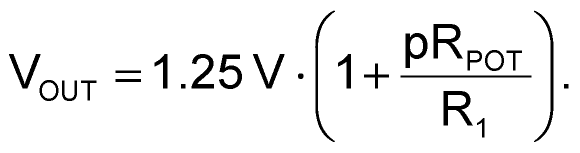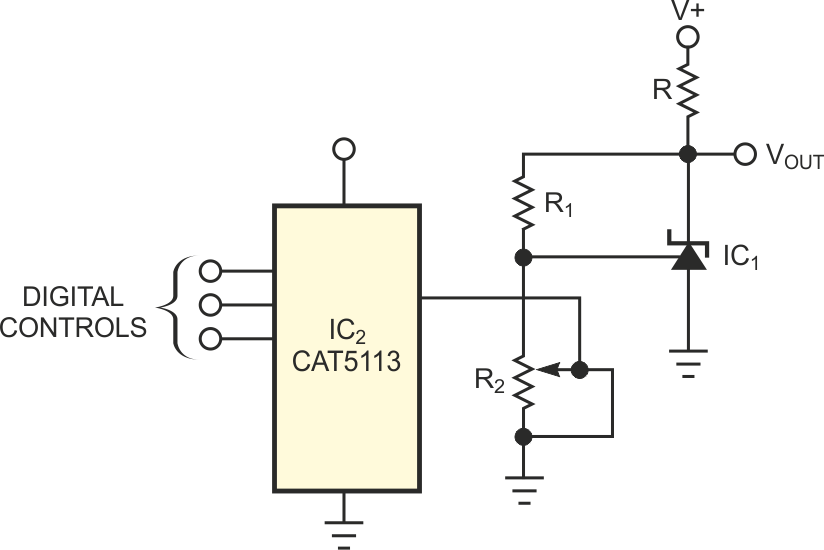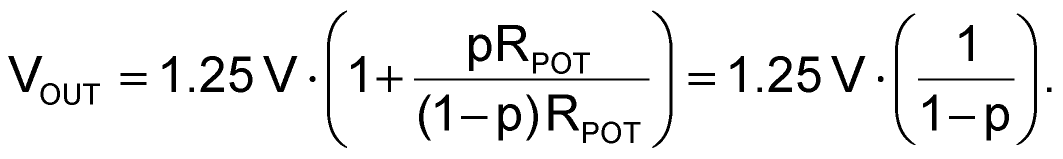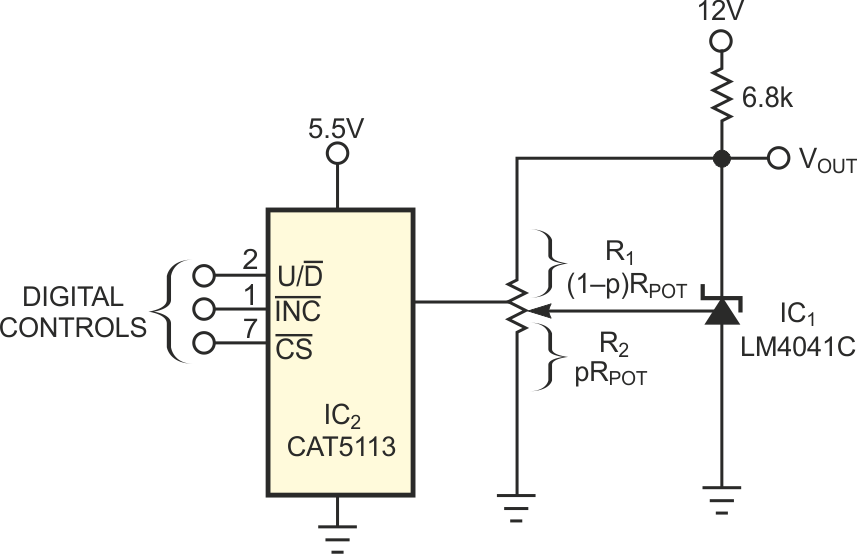# Digital potentiometer programs and stabilizes voltage reference

## ON Semiconductor CAT5113

Chuck Wojslaw

EDN

The potentiometer portion of a mixed-signal, digitally programmable potentiometer adds variability to an analog circuit, and its digital controls provide programmability. You can use a digital potentiometer in two ways in an analog circuit. You can use it as a two-terminal variable resistance, or rheostat, or as a three-terminal resistive divider. Although both ways bring variability to the analog circuit, the three-terminal implementation usually brings other important characteristics as well. For example, a programmable voltage reference has two implementations. The circuit in Figure 1 is a voltage reference whose output, VOUT, depends on the 1.25 V reference of the shunt regulator, IC1, R1, and the programmable resistance R2 = pRPOT. The value of p varies from 0 to 1; it represents the proportional setting of the wiper from one end, 0, to the other, 1. For this circuit,As p varies from 0 to 1, VOUT varies from 1.25 V to some maximum value established by R1 and the potentiometer's end-to-end resistance, RPOT. The temperature coefficient of VOUT is proportional to those of the LM4041CIZ regulator's 1.25 V reference, R1, and RPOT. The temperature coefficient of the reference voltage and a quality resistor are lower than 100 ppm/°C. However, the temperature coefficient of RPOT is not guaranteed and can run in the hundreds of parts per million per degrees Celsius. Thus, the temperature stability of RPOT has adverse effects on the temperature coefficient of VOUT.Figure 1. In this circuit, the potentiometer’s temperature coefficient adversely affects that of VOUT.

The programmable voltage-reference circuit in Figure 2 uses the potentiometer as a three-terminal device. For this circuit,This implementation shifts the temperature dependence of VOUT on the potentiometer from the high temperature coefficient of RPOT to the potentiometer's low ratiometric temperature coefficient of 20 ppm/°C. It also reduces component count and cost and increases programming accuracy. The 15% accuracy of RPOT is the dominant factor in the accuracy of VOUT in the circuit of Figure 1. In the circuit of Figure 2, the potentiometer's 1% linearity is the dominant factor in the accuracy of VOUT. For the values shown in Figure 2, the 100-tap CAT5113 digitally programmable potentiometer provides for a variable, temperature-stable VOUT of 1.25 to 5.5 V (0 ≤ p ≤ 0.77). The measured data correlates to better than 1% with the calculated values.Figure 2. This circuit has low component count, low temperature coefficient, and enhanced programming accuracy.

## Materials on the topic

EDN